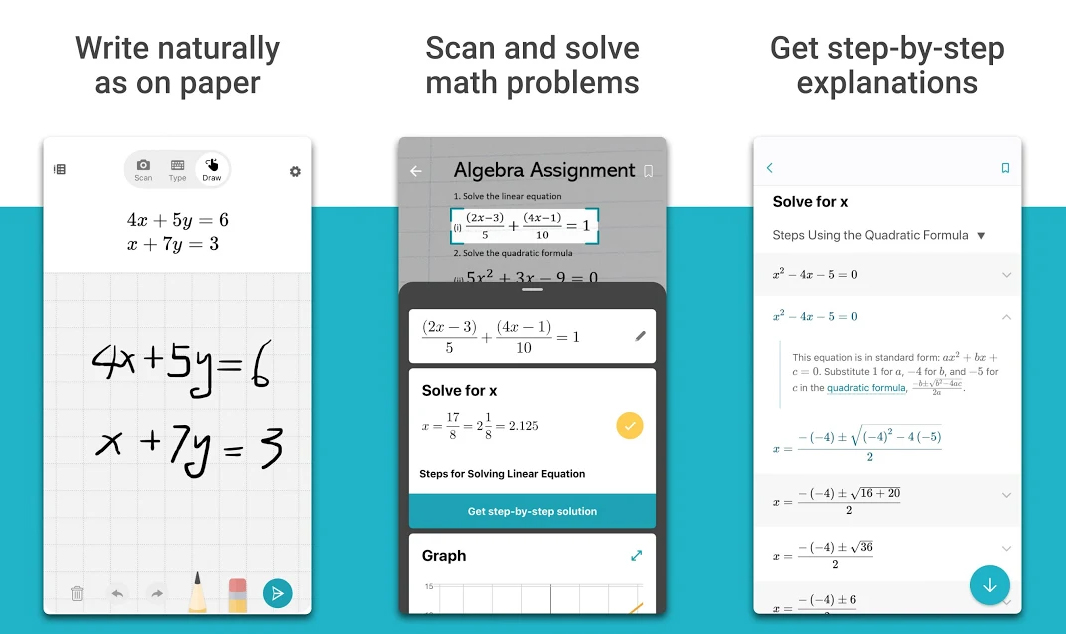Even if you know you need math in the future and even if you love it, sometimes you just can’t stand it. All those formulas and theorems sometimes get in your nerves and you just snap. Back in my day, we hand no choice but to calm ourselves and go back to solving the problem. Fortunately, you guys have a choice. If you can’t get around solving a math problem, you can use Microsoft’s Math Solver. That’s right, you have an app that can solve math for you. Here’s how to solve math using Android.

## Solve Math using Android

Microsoft’s Math Solver helps you with tons of problems that you may find difficult. You can use the app to solve arithmetic, algebra, calculus, trigonometry, statistics, pre-algebra and much more. Using an AI-powered math solver, this app can handle most of the problems you throw at it. Although during testing, we didn’t throw anything too crazy at it, since there are different ways it can solve a problem, we are pretty sure it can handle a little crazy. Get Microsoft’s Math Solver for free from the Google Play Store.There already are apps that can solve math problems, but we haven’t seen anything as advanced as Microsoft’s Math Solver. You can take a photo of the problem, type it out or even draw it on the screen; the app will solve it. The app will recognize the problem, solve it and provide step by step explanation to the problem. If the app can’t solve a problem, it will use Bing to help you find the solution. Add Bing to the list of features and you have an app that can solve almost every math problem you provide it.

Here are the features that you get when using Microsoft’s Math Solver;

• Write a math problem on the screen to solve.
• Take a photo of a printed or written questions.
• Type the problem using the advanced calculator.
• Get step by step explanation for better understanding.
• Import images of math equations that you’ve already taken.
• Search the web of similar questions.
• Search for video lectures.
• Test yourself with math word problems.
• Scan and plot x-y data tables for linear and non-linear functions.
• Learn math in your language. Math Solver supports many languages including Chinese, Hindi, Spanish, Russian and much more.

We do not recommend you use this app for each and every problem. You need to learn too. Use this app if you really need it and when you are struggling with certain math problems.